NCERT Solutions for Class 9 Maths Chapter 4 Lines and Angles Ex 4.3 are part of NCERT Solutions for Class 9 Maths. Here we have given NCERT Solutions for Class 9 Maths Chapter 4 Lines and Angles Ex 4.3.

 Board CBSE Textbook NCERT Class Class 9 Subject Maths Chapter Chapter 4 Chapter Name Lines and Angles Exercise Ex 4.3 Number of Questions Solved 6 Category NCERT Solutions

## NCERT Solutions for Class 9 Maths Chapter 4 Lines and Angles Ex 4.3

Question 1.
In figure, sides QP and RQ of APQR are produced to points S and T, respectively. If ∠SPR = 135° and ∠PQT = 110°, find ∠PRQ.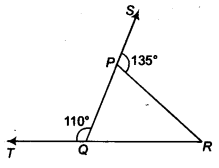Solution: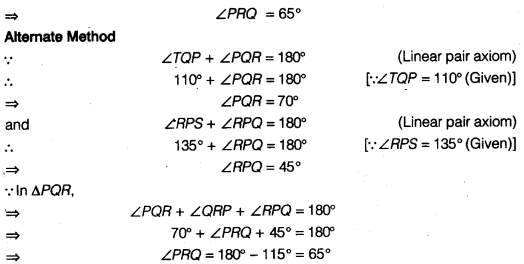Question 2.
In figure, ∠X – 62°, ∠XYZ = 54°, if YO and ZO are the bisectors of ∠XYZ and ∠XZY respectively of ∆XYZ, find ∠OZY and ∠YOZ.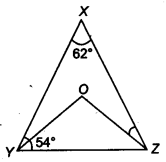Solution:
In ∆XYZ,
∵ ∠X+ ∠Y+ ∠Z = 180°
(Sum of all angles of triangle is equal to
∴ 62° + ∠Y + ∠Z = 180° [YZX = 62° (Given)]
⇒ ∠Y + ∠Z = 118° .
⇒ $$\frac { 1 }{ 2 }$$∠Y + $$\frac { 1 }{ 2 }$$ ∠Z = $$\frac { 1 }{ 2 }$$ x 118°
⇒ ∠OYZ + ∠OZY = 59°
(∵ YO and ZO are the bisectors of ∠XYZ and ∠XZY)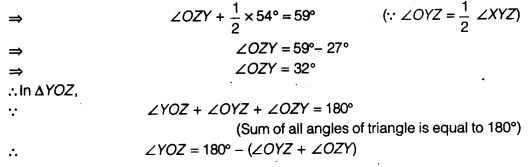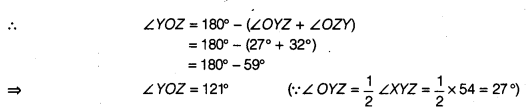Question 3.
In figure, if AB || DE, ∠BAC = 35° and ∠CDE = 539 , find ∠DCE.Solution:
We have AB || DE
⇒ ∠AED= ∠ BAE (Alternate interior angles)
Now, ∠ BAE = ∠ BAC
⇒ ∠ BAE = 35° [ ∵ ∠ BAC = 35° (Given) ]
∴ ∠ AED = 35°
In ∆DCE,
∵ ∠DCE + ∠CED+ ∠EDC= 180° ( ∵Sum of all angles of triangle is equal to 180°)
⇒ ∠ DCE + 35°+ 53° = 180° ( ∵∠ AED = ∠ CED = 35°)
⇒ ∠ DCE = 180° – (35° + 53°)
⇒ ∠ DCE = 92°

Question 4.
In figure, if lines PQ and RS intersect at point T, such that ∠ PRT = 40°, ∠ RPT = 95° and ∠TSQ = 75°, find ∠ SQT.Solution:
∵∠PTS = ∠RPT + ∠PRT (Exterior angle = Sum of interior opposite angles)
∠ PTS = 95° + 40° [∵ ∠PPT = 95° (Given)]
⇒ ∠ PTS = 135° [and ∠PRT = 40°]
Also, ∠ TSQ + ∠ SQT = ∠ PTS (Exterior angle = Sum of interior opposite angles)
⇒ 75°+ ∠ SQT = 135°
⇒ ∠ SQT = 60° [∵ ∠ TSQ = 75° (Given)]

Question 5.
In figure, if PQ ⊥ PS, PQ||SR, ∠SQR = 2S° and ∠QRT = 65°, then find the values of x and y.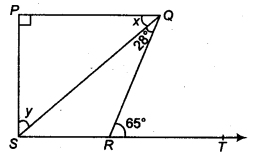Solution:
Here, PQ || SR
⇒ ∠ PQR = ∠ OPT (Alternate interior angles)
⇒ x + 28° = 65° ⇒ x = 37°
Now, in right angled ASPQ, we have ∠P = 90°
∴ ∠P + x + y = 180° (∵ Sum of all angles of a triangle is equal to 180°)
⇒ 90°+ 37°+ y= 180°
⇒ 127°+ y=180°
⇒ y = 53°

Question 6.
In figure, the side QR of A PQR is produced to a point S. If the bisectors of ∠PQR and ∠PRS meet at point T, then prove thatSolution:
In ∆ PQP,
∵ ∠QPR + ∠PQR = ∠PRS …(i)
(∵ Sum of interior opposite angles = Exterior angle)
Now, in ∆ TOR,
∵ ∠QTR + ∠TQR = ∠TRS …..(ii)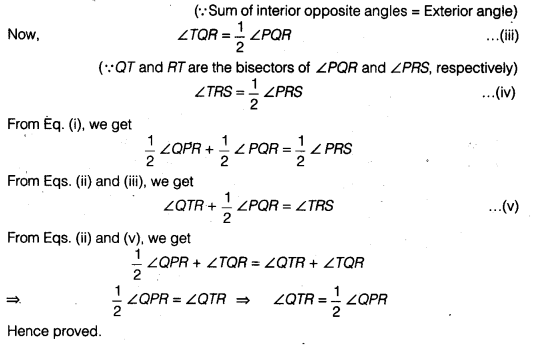We hope the NCERT Solutions for Class 9 Maths Chapter 4 Lines and Angles Ex 4.3 help you. If you have any query regarding NCERT Solutions for Class 9 Maths Chapter 4 Lines and Angles Ex 4.3, drop a comment below and we will get back to you at the earliest.